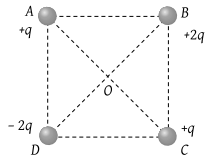An electric dipole is in unstable equilibrium in the uniform electric field. The angle between its dipole moment and the electric field is

1.  90$°$

2.  120$°$

3.  0$°$

4.  180$°$

Subtopic:  Electric Dipole |
To view explanation, please enrol in the course below.
NEET 2021 - Achiever Batch - Aryan Raj Singh

Difficulty Level:

A particle having charge ${\mathrm{q}}_{1}$ exerts F electrostatic force on charge ${\mathrm{q}}_{2}$ at rest. If a particle having charge $\frac{{q}_{1}}{4}$ is placed midway between the line joining the two charges  then electrostatic force on ${\mathrm{q}}_{2}$ due to ${\mathrm{q}}_{1}$ will become/remain

1. 2F

2. $\frac{F}{2}$

3. F

4. zero

Subtopic:  Coulomb's Law |
To view explanation, please enrol in the course below.
NEET 2021 - Achiever Batch - Aryan Raj Singh

Difficulty Level:

A charge q is to be divided into two small conducting spheres. What should be the value of charges on the spheres so that when placed at a certain distance apart, the repulsive force between them is maximum?

(1)

(2)

(3)

(4)

Subtopic:  Coulomb's Law |
To view explanation, please enrol in the course below.
NEET 2021 - Achiever Batch - Aryan Raj Singh

Difficulty Level:

You are traveling in a car during a thunderstorm. In order to protect yourself from lightning, you would prefer to

(1) Touch the nearest electric pole

(2) Take shelter under a tree

(3) Get out and crouch on the ground

(4) Remain in the car

Subtopic:  Electric Field |
To view explanation, please enrol in the course below.
NEET 2021 - Achiever Batch - Aryan Raj Singh

Difficulty Level:

If a soap bubble is given some charge, then its radius

(1) Increases

(2) Decreases

(3) Remain unchanged

(4) May increase or decrease depending upon the give charge is positive or negative

Subtopic:  Ways of Charging |
To view explanation, please enrol in the course below.
NEET 2021 - Achiever Batch - Aryan Raj Singh

Difficulty Level:

An electric dipole of dipole moment p is placed in an electric field of intensity E such that angle between electric field and dipole moment is $\theta$. Assuming that the potential energy of the dipole is zero when $\theta =0°$, the potential energy of the dipole will be

(1) -pE cos$\theta$

(2) pE(1-cos$\theta$)

(3) pE(cos$\theta$-1)

(4) -2pE(cos$\theta$-1)

Subtopic:  Electric Dipole |
To view explanation, please enrol in the course below.
NEET 2021 - Achiever Batch - Aryan Raj Singh

Difficulty Level:

The electrostatic field due to a charged conductor just outside the conductor is

1. zero and parallel to the surface at every point inside the conductor

2. zero and is normal to the surface at every point inside the conductor

3. parallel to the surface at every point and zero inside the conductor

4. normal to the surface at every point and zero inside the conductor

Subtopic:  Electric Field |
To view explanation, please enrol in the course below.
NEET 2021 - Achiever Batch - Aryan Raj Singh

Difficulty Level:

The law, governing the force between electric charges is known as

(1) Ampere's law

(2) Ohm's law

(3) Faraday's law

(4) Coulomb's law

Subtopic:  Coulomb's Law |
To view explanation, please enrol in the course below.
NEET 2021 - Achiever Batch - Aryan Raj Singh

Difficulty Level:

Fg and Fe represents gravitational and electrostatic force respectively between electrons situated at a distance 10 cm. The ratio of Fg/ Fe is of the order of

(1) 1042

(2) 10

(3) 1

(4) 10–43

Subtopic:  Coulomb's Law |
To view explanation, please enrol in the course below.
NEET 2021 - Achiever Batch - Aryan Raj Singh

Difficulty Level:

Four charges are arranged at the corners of a square ABCD, as shown in the adjoining figure. The force on the charge kept at the centre O is(1) Zero

(2) Along the diagonal AC

(3) Along the diagonal BD

(4) Perpendicular to side AB

Subtopic:  Coulomb's Law |
To view explanation, please enrol in the course below.
NEET 2021 - Achiever Batch - Aryan Raj Singh

Difficulty Level: